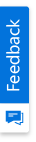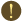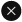We use cookies to give you the best experience on our website. If you continue to browse, then you agree to our privacy policy and cookie policy.Unfortunately, activation email could not send to your email. Please try again.# Why the diagram prints four pages by default? How can I print the diagram in a single page?

Platform: WinForms |
Control: Diagram

## Print diagram in single page

The Essential Diagram printing implementation uses the size of your diagram model and the printer page setting for calculating the number of pages to be rendered. Even though you might have only one page worth of nodes in your diagram model, if the model bounds are larger, the diagram control will attempt to paginate and print the entire model.

To print the diagram in a single page, you have to temporarily modify the size of model.

C#

```// The desired page size is 21 x 29.7 centimeters
// The margins are of size 1 inch or 25 mm
int verticalMargin = 260;
int horizontalMargin = 260;
// The following units are in millimeters, so convert them to pixels
float pageHeight = Diagram.MeasureUnitsConverter.Convert((2970 - (verticalMargin * 2)) / 10, MeasureUnits.Millimeter, MeasureUnits.Pixel);
// float pageHeight = bounds.Height;
float pageWidth = Diagram.MeasureUnitsConverter.Convert((2100 - (horizontalMargin * 2)) / 10, MeasureUnits.Millimeter, MeasureUnits.Pixel);
// Set the model height to twice the page height
diagram1.Model.DocumentSize.Height = (int)pageHeight / 2;
// Set the model width to page width
diagram1.Model.DocumentSize.Width = (int)pageWidth;
this.PrintPreview( );

```

VB

```// The desired page size is 21 x 29.7 centimeters
// The margins are of size 1 inch or 25 mm
Dim verticalMargin As Integer = 260
Dim horizontalMargin As Integer = 260
// The following units are in millimeters, so convert them to pixels
Dim pageHeight As Single = Diagram.MeasureUnitsConverter.Convert((2970 - (verticalMargin * 2)) / 10, MeasureUnits.Millimeter, MeasureUnits.Pixel)
// float pageHeight = bounds.Height;
Dim pageWidth As Single = Diagram.MeasureUnitsConverter.Convert((2100 - (horizontalMargin * 2)) / 10, MeasureUnits.Millimeter, MeasureUnits.Pixel)
' Set the model height to twice the page height
diagram1.Model.Size = New SizeF(pageWidth, pageHeight / 2)
Me.PrintPreview( )
```

Please refer to the attached sample that illustrates this.

http://help.syncfusion.com/support/samples/kb/Diagram.Windows/Diagram_WF_Printing/Diagram_WF_Printing.zip

2X faster development

The ultimate WinForms UI toolkit to boost your development speed.You are using an outdated version of Internet Explorer that may not display all features of this and other websites. Upgrade to Internet Explorer 8 or newer for a better experience.